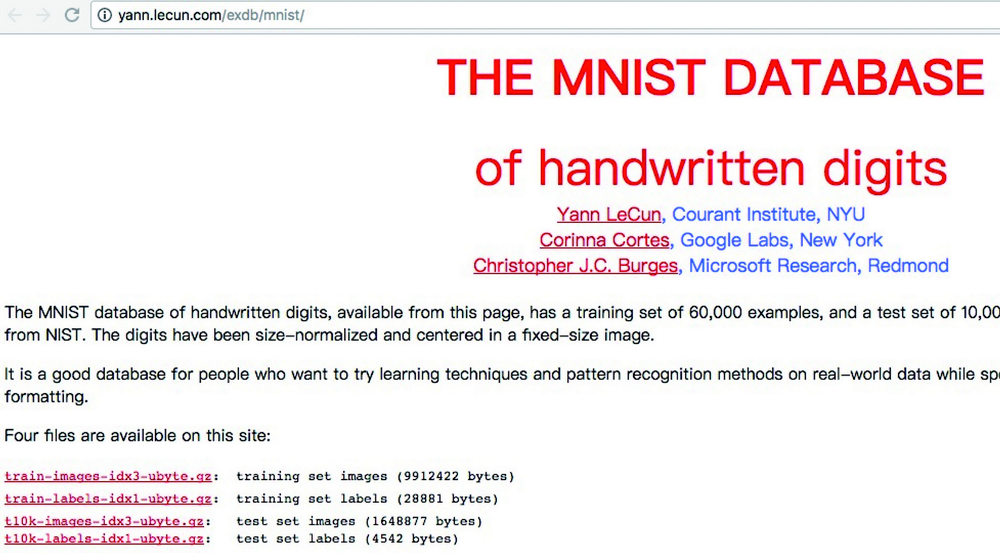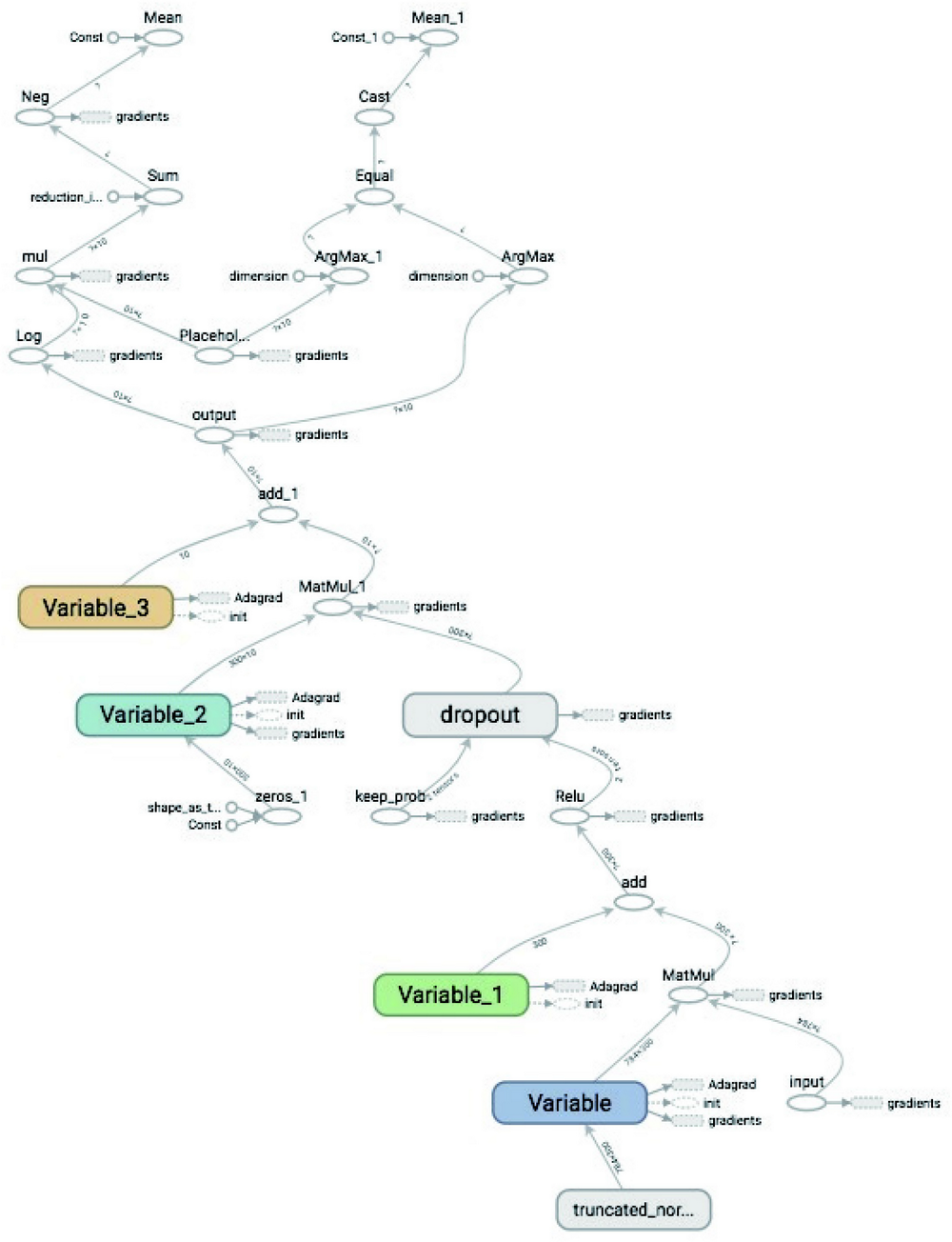# AI 安全之对抗样本入门 (33)：打造对抗样本工具箱 3.2(TensorFlow)

TensorFlow 是被工业界和学术界使用最广泛的深度学习框架之一。我们以解决经典的手写数字识别的问题为例，介绍 TensorFlow 的基本使用方法，代码路径为：

https://github.com/duoergun0729/adversarial_examples/blob/master/code/2-tensorflow.ipynb
1. 加载相关库

import tensorflow as tffrom tensorflow.examples.tutorials.mnist import input_datafrom tensorflow.python.framework import graph_utilimport os
1. 加载数据集

MNIST 是一个入门级的计算机视觉数据集，它包含各种手写数字图片如图 3-3 所示。train-images-idx3-ubyte.gz 60000 个图片训练样本
train-labels-idx1-ubyte.gz 60000 个图片训练样本的标注
t10k-images-idx3-ubyte.gz 10000 个图片测试样本
t10k-labels-idx1-ubyte.gz 10000 个图片测试样本的标注

MNIST 的网址为： http://yann.lecun.com/exdb/mnist/ 。MNIST 官网如图 3-4 所示。MNIST 默认图像的形状为 [28,28,1]，为了便于处理，需要改变其形状为一维向量 784。另外特征数据需要归一化为 0 到 1，这可以通过除以 255 来完成。默认的标签的数据类型为整数，取值范围为 0 到 9，为了便于深度学习网络训练，通常会把标签数据转换为独热编码 (One Hot)。所谓独热编码，又称一位有效编码，其方法是使用 N 位状态寄存器来对 N 个状态进行编码，每个状态都有它独立的寄存器位，并且在任意时候，其中只有一位有效。比如标签 0 到 5 就可以分别编码为：

000001,000010,000100,001000,010000,100000
1. 定义网络结构in_units = 784 #输入节点数h1_units = 300 #隐藏层节点数#初始化隐藏层权重 W1，服从默认均值为 0，标准差为 0.1 的截断正态分布W1 = tf.Variable(tf.truncated_normal([in_units, h1_units], stddev=0.1))b1 = tf.Variable(tf.zeros([h1_units])) #隐藏层偏置 b1 全部初始化为 0W2 = tf.Variable(tf.zeros([h1_units, 10]))b2 = tf.Variable(tf.zeros())x = tf.placeholder(tf.float32, [None, in_units],name="input")keep_prob = tf.placeholder(tf.float32,name="keep_prob")#定义模型结构hidden1 = tf.nn.relu(tf.matmul(x, W1) + b1)hidden1_drop = tf.nn.dropout(hidden1, keep_prob)y = tf.nn.softmax(tf.matmul(hidden1_drop, W2) + b2,name="output")
1. 定义损失函数和优化器

y_ = tf.placeholder(tf.float32, [None, 10])cross_entropy = tf.reduce_mean(-tf.reduce_sum(y_ * tf.log(y),reduction_indices=))train_step = tf.train.AdagradOptimizer(0.3).minimize(cross_entropy)
1. 训练与验证

sess.run(tf.global_variables_initializer())correct_prediction = tf.equal(tf.arg_max(y, 1), tf.arg_max(y_, 1))accuracy = tf.reduce_mean(tf.cast(correct_prediction, tf.float32))for i in range(5000):     batch_xs, batch_ys = mnist.train.next_batch(100)     _,loss=sess.run([train_step,cross_entropy],{x: batch_xs, y_: batch_ys, keep_prob: 0.75})     if i % 200 == 0:           acc=accuracy.eval(feed_dict={x:mnist.test.images,                                         y_:mnist.test.labels,keep_prob:1})            print("loss={},acc={}".format(loss,acc))

loss=0.021222606301307678,acc=0.9814000129699707loss=0.04722728207707405,acc=0.9786999821662903loss=0.024759886786341667,acc=0.9797000288963318loss=0.009720790199935436,acc=0.9803000092506409